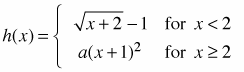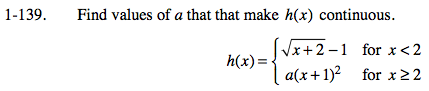### Home > CALC > Chapter 1 > Lesson 1.4.1 > Problem1-139

1-139.
1. Find values of a that that make h(x) continuous. Homework Help ✎If the function is continuous, both pieces of h(x) will be connected at x = 2. That means that both pieces will have the same y-value at x = 2.

Equate both parts of the function for x = 2. Then solve for a.

$\sqrt{2+2}-1=a(2+1)^2$

$2-1=9a$